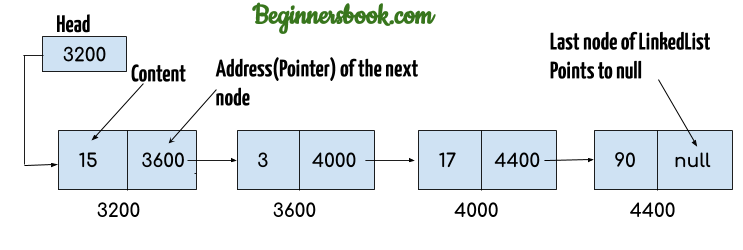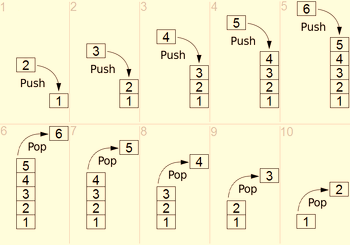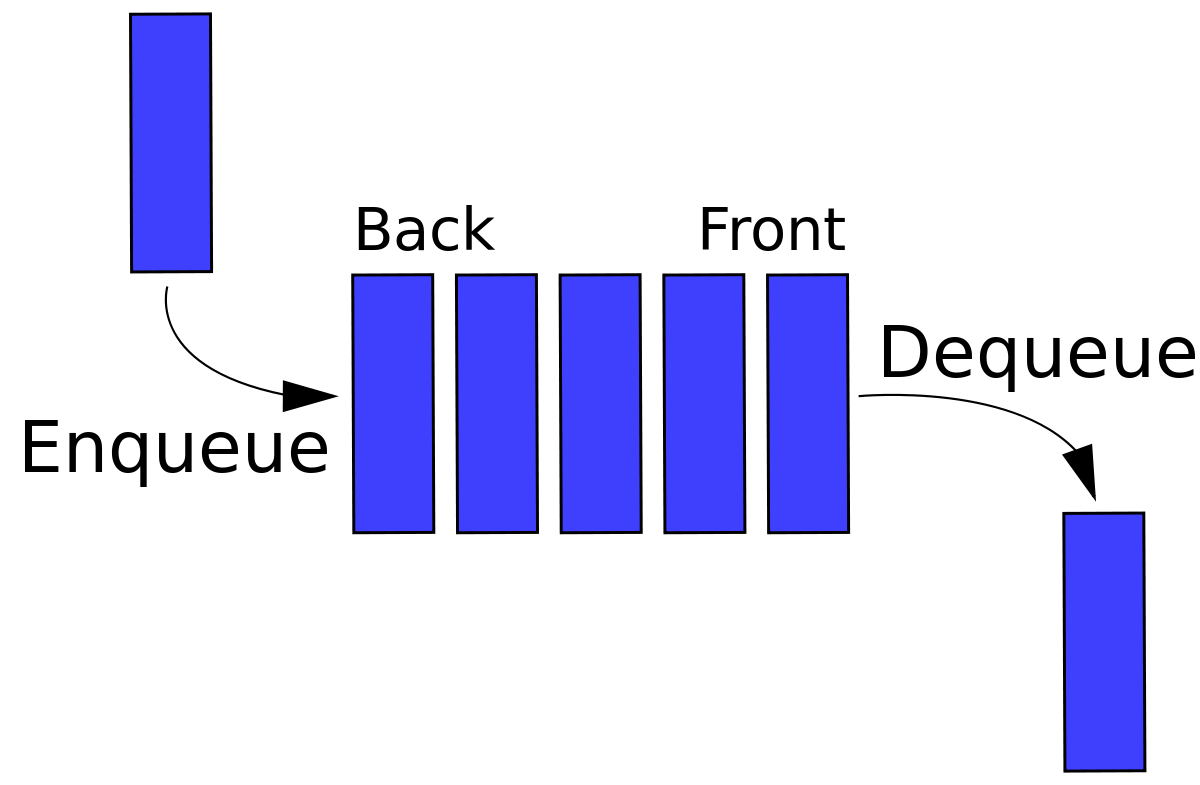# Pointers and Abstract Datatype

## Basic definitions:

• Address of a memory: a memory address is an exact assigned location in RAM used to track where information is stored
• Pointer: a pointer is a variable that holds a memory address of an object in memory.
• The address is the location of another object in the memory
• Pointer is something that points or indicates a figure or a position
• Not all pointers contain an address example: Null pointer
• The value of a null pointer is 0
• Note that pointer contain memory address not data values

## Pointer declaration

• The syntax of declaring a pointer in programming is

Datatype *pointer_variable

e.g. Int *p;

the Asterix * is used to signify the variable as a pointer variable

## Pointer operators

• The “&” operator is a unary operator that returns the memory address of it operand e.g. if x is a variable, then the &x should be read as “the address of x”

### Indirection operator (*)

• The * operator is a unary that returns the value of the variable located at the address specialized by the operand.

Example

#include <stdio.h>

int main() {

int x = 850;

int *ptr;

ptr = &x;

printf(“i am Franklin The Situation demonstrating pointers\n”);

printf(“the address of variable x = %p\n”, &x);

printf(“the value stored in address %p = %d\n”,ptr,*ptr);

}

}## Application of pointers: LINKED LIST

• A linked list is a linear data structure
• Nodes make up linked list
• Nodes are structures made up of data and a pointer to another node
• The pointer is called next

## Operation of a linked list

• Insertions operation: insertion can be done at any position. To determine where the element is to be placed, we will require a searching method.
• Deletion operation: to delete a data item as well, we will need a searching method to locate the position of the item.

A linked list can be used to

• implement a stack and a queue
• implementation of graphs
• dynamic memory allocation
• performing mathematical operations on long mathematical operation
• manipulation of polynomials by storing contents in a node of a linked list
• representing sparse metrices.Illustration of a pointer

# ABSTRACT DATA TYPE

To process data types in a computer, we need to define the data type and operations (+, -, *) to be performed on the data.

Datatype + operations = Abstract Datatype (ADT)

Abstract Data type means to hide how operations are being performed on the data (we cant see it). There are Two categories of abstract data type

• Linear ADT (vector, queue, stack etc.)

An abstract data type should provide a way to

• Remove data items
• Find, retrieve or access an item

## Stack

• A stack is a restricted data structure in which all additions and deletions are made at one end (the top)
• Items inserted into a stack are removed in a reversed order. The reversing order is termed (LIFO) Last In First Out data structure. E.g. a stack of blocks, plates classification, books.

### Operation of stack

There are basically 2 types of stack operation

• PUSH (adding items in a stack)
• POP (removing items from a stack or deleting contents from a stack)Operation of a stack

## Queue

• This is a linear list in which data can only be inserted at one end called the rear and deleted from the other end called the
• Items inserted into a stack are removed in thesame order. This order is termed is termed (FIFO) First In First Out data structure.

### Operation of a queue

There are basically two queue operation which are

• Enqueue operation (inserting or adding data items)
• Dequeue operation (removing or deleting data items)Operation of a queue

# A tree

A tree is another data structure that you can use to store information. Unlike stacks and queues, which are linear data structures, trees are hierarchical data structures

The element at the top of the tree is called the root. The elements that are directly under an element are called its children